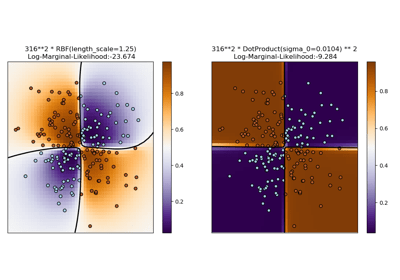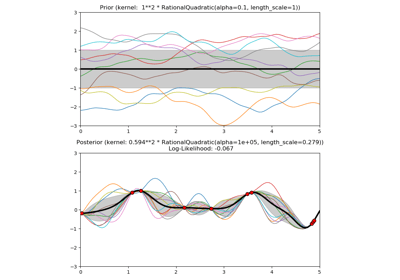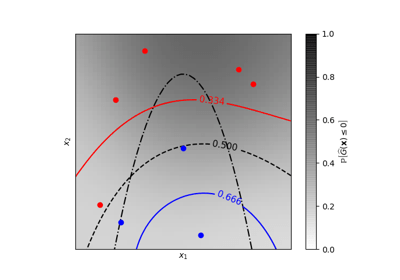/scikit-learn

# sklearn.gaussian_process.kernels.DotProduct

`class sklearn.gaussian_process.kernels.DotProduct(sigma_0=1.0, sigma_0_bounds=(1e-05, 100000.0))` [source]

Dot-Product kernel.

The DotProduct kernel is non-stationary and can be obtained from linear regression by putting N(0, 1) priors on the coefficients of x_d (d = 1, . . . , D) and a prior of N(0, sigma_0^2) on the bias. The DotProduct kernel is invariant to a rotation of the coordinates about the origin, but not translations. It is parameterized by a parameter sigma_0^2. For sigma_0^2 =0, the kernel is called the homogeneous linear kernel, otherwise it is inhomogeneous. The kernel is given by

k(x_i, x_j) = sigma_0 ^ 2 + x_i cdot x_j

The DotProduct kernel is commonly combined with exponentiation.

New in version 0.18.

Parameters: `sigma_0 : float >= 0, default: 1.0` Parameter controlling the inhomogenity of the kernel. If sigma_0=0, the kernel is homogenous. `sigma_0_bounds : pair of floats >= 0, default: (1e-5, 1e5)` The lower and upper bound on l `bounds` Returns the log-transformed bounds on the theta. hyperparameter_sigma_0 `hyperparameters` Returns a list of all hyperparameter specifications. `n_dims` Returns the number of non-fixed hyperparameters of the kernel. `theta` Returns the (flattened, log-transformed) non-fixed hyperparameters.

#### Methods

 `__call__`(X[, Y, eval_gradient]) Return the kernel k(X, Y) and optionally its gradient. `clone_with_theta`(theta) Returns a clone of self with given hyperparameters theta. `diag`(X) Returns the diagonal of the kernel k(X, X). `get_params`([deep]) Get parameters of this kernel. `is_stationary`() Returns whether the kernel is stationary. `set_params`(**params) Set the parameters of this kernel.
`__init__(sigma_0=1.0, sigma_0_bounds=(1e-05, 100000.0))` [source]
`__call__(X, Y=None, eval_gradient=False)` [source]

Return the kernel k(X, Y) and optionally its gradient.

Parameters: `X : array, shape (n_samples_X, n_features)` Left argument of the returned kernel k(X, Y) `Y : array, shape (n_samples_Y, n_features), (optional, default=None)` Right argument of the returned kernel k(X, Y). If None, k(X, X) if evaluated instead. `eval_gradient : bool (optional, default=False)` Determines whether the gradient with respect to the kernel hyperparameter is determined. Only supported when Y is None. `K : array, shape (n_samples_X, n_samples_Y)` Kernel k(X, Y) `K_gradient : array (opt.), shape (n_samples_X, n_samples_X, n_dims)` The gradient of the kernel k(X, X) with respect to the hyperparameter of the kernel. Only returned when eval_gradient is True.
`bounds`

Returns the log-transformed bounds on the theta.

Returns: `bounds : array, shape (n_dims, 2)` The log-transformed bounds on the kernel’s hyperparameters theta
`clone_with_theta(theta)` [source]

Returns a clone of self with given hyperparameters theta.

Parameters: `theta : array, shape (n_dims,)` The hyperparameters
`diag(X)` [source]

Returns the diagonal of the kernel k(X, X).

The result of this method is identical to np.diag(self(X)); however, it can be evaluated more efficiently since only the diagonal is evaluated.

Parameters: `X : array, shape (n_samples_X, n_features)` Left argument of the returned kernel k(X, Y) `K_diag : array, shape (n_samples_X,)` Diagonal of kernel k(X, X)
`get_params(deep=True)` [source]

Get parameters of this kernel.

Parameters: `deep : boolean, optional` If True, will return the parameters for this estimator and contained subobjects that are estimators. `params : mapping of string to any` Parameter names mapped to their values.
`hyperparameters`

Returns a list of all hyperparameter specifications.

`is_stationary()` [source]

Returns whether the kernel is stationary.

`n_dims`

Returns the number of non-fixed hyperparameters of the kernel.

`set_params(**params)` [source]

Set the parameters of this kernel.

The method works on simple kernels as well as on nested kernels. The latter have parameters of the form `<component>__<parameter>` so that it’s possible to update each component of a nested object.

Returns: self
`theta`

Returns the (flattened, log-transformed) non-fixed hyperparameters.

Note that theta are typically the log-transformed values of the kernel’s hyperparameters as this representation of the search space is more amenable for hyperparameter search, as hyperparameters like length-scales naturally live on a log-scale.

Returns: `theta : array, shape (n_dims,)` The non-fixed, log-transformed hyperparameters of the kernel

## Examples using `sklearn.gaussian_process.kernels.DotProduct`Illustration of Gaussian process classification (GPC) on the XOR datasetIllustration of prior and posterior Gaussian process for different kernelsIso-probability lines for Gaussian Processes classification (GPC)

© 2007–2018 The scikit-learn developers